# How do I approach this? 61. Use Euler's method to find approximate values for the solution...

How do I approach this?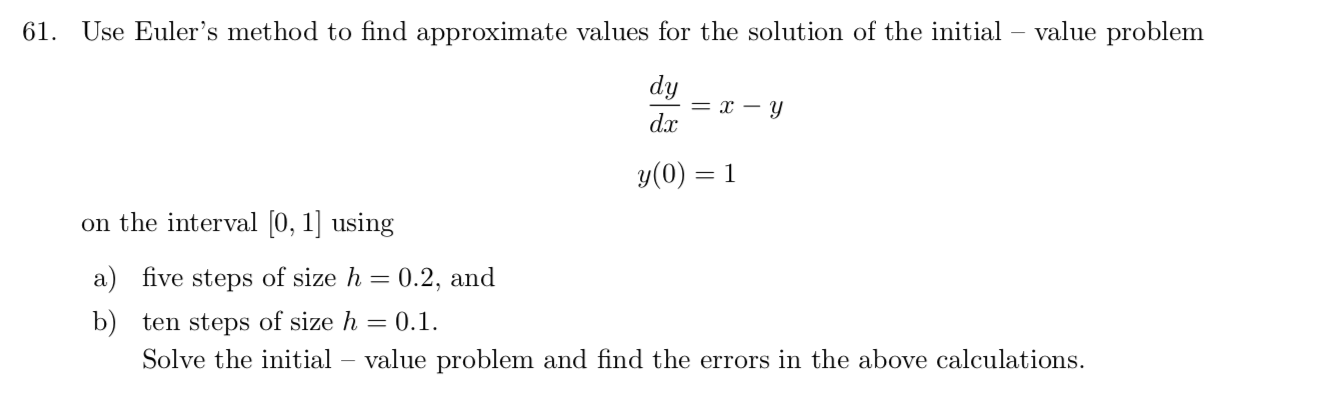61. Use Euler's method to find approximate values for the solution of the initial value problem dy dx = I – Y y(0) 1 on the interval [0, 1] using a) five steps of size h = 0.2, and b) ten steps of size h = 0.1. Solve the initial – value problem and find the errors in the above calculations.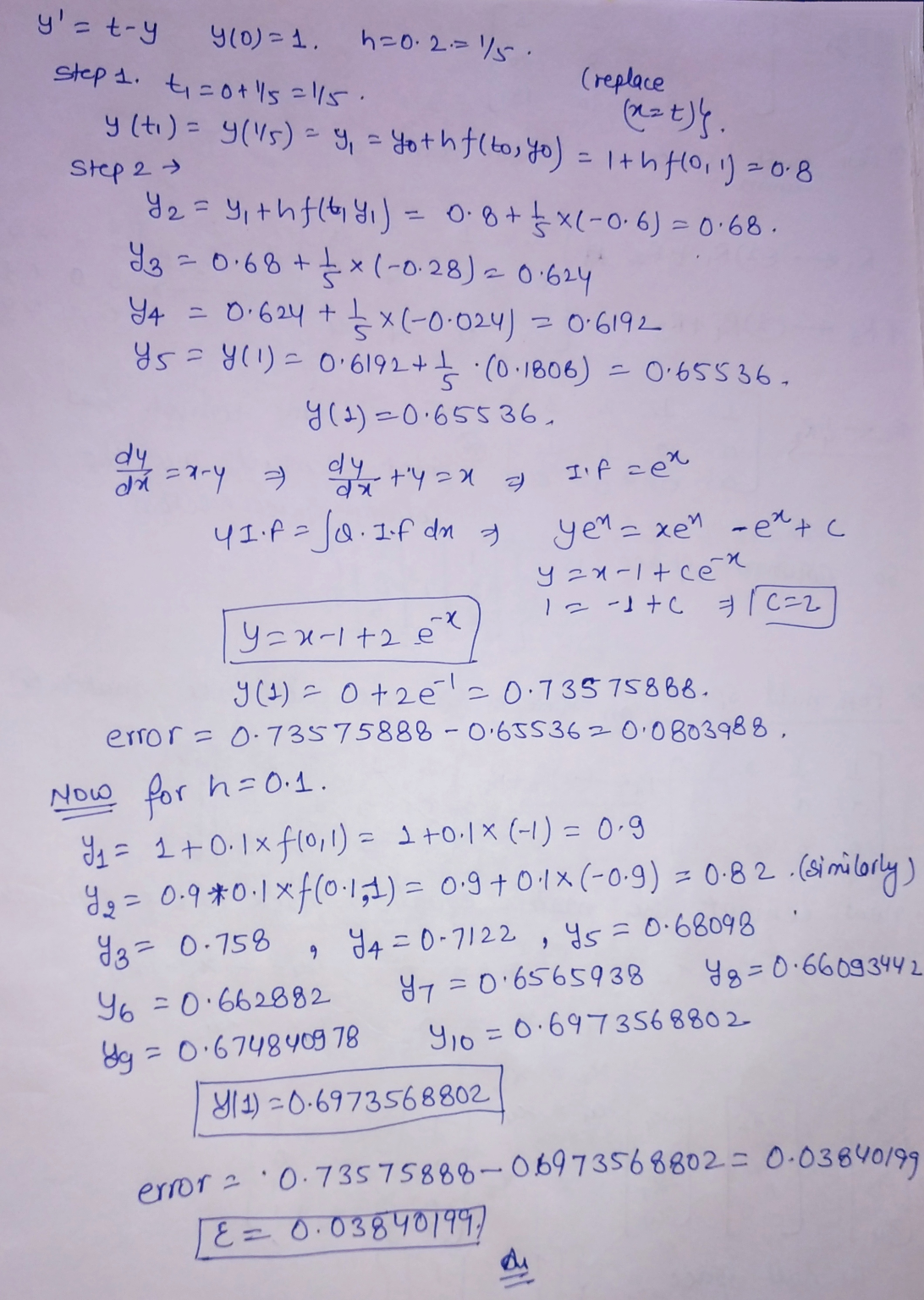##### Add Answer of: How do I approach this? 61. Use Euler's method to find approximate values for the solution...
Similar Homework Help Questions
• ### dy Use Euler's Method with step size h = 0.2 to approximate y(1), where y(x) is...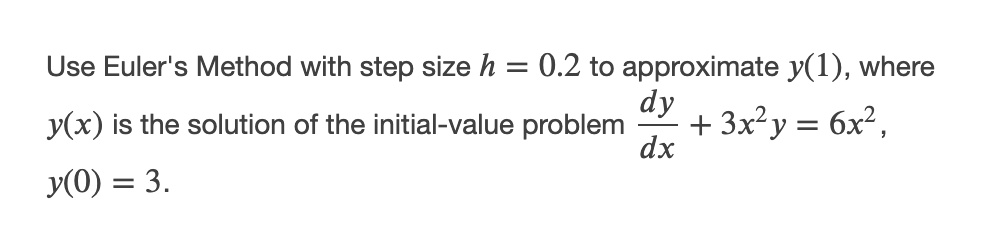dy Use Euler's Method with step size h = 0.2 to approximate y(1), where y(x) is the solution of the initial-value problem + 3x2y = 6x2, dx y(0) = 3.

• ### Euler's method

dy/dx=x2+y2Use Euler's method to find approximate value of a solution. if y(0)=1, use Euler's method with step size 0.1 to approximate y(0.2)"Show all work please"

• ### 3. Euler's Method (a) Use Euler's Method with step size At = 1 to approximate values...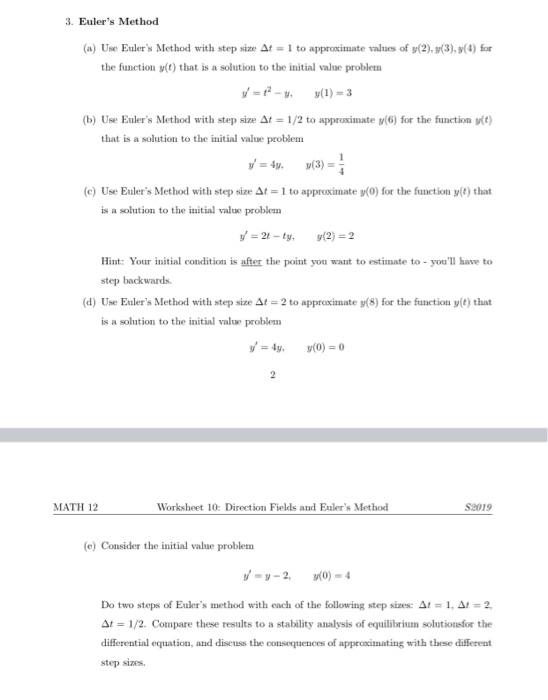3. Euler's Method (a) Use Euler's Method with step size At = 1 to approximate values of y(2),3(3), 3(1) for the function y(t) that is a solution to the initial value problem y = 12 - y(1) = 3 (b) Use Euler's Method with step size At = 1/2 to approximate y(6) for the function y(t) that is a solution to the initial value problem y = 4y (3) (c) Use Euler's Method with step size At = 1 to...

• ### I. Use Euler's method with step size h = 0.1 to numerically solve the initial value problem y,--2ty+y2, y(0) 1 on the interval 0 < t 2. Compare your approximations with the exact solution....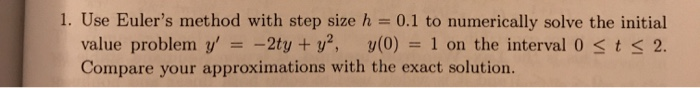I. Use Euler's method with step size h = 0.1 to numerically solve the initial value problem y,--2ty+y2, y(0) 1 on the interval 0 < t 2. Compare your approximations with the exact solution. I. Use Euler's method with step size h = 0.1 to numerically solve the initial value problem y,--2ty+y2, y(0) 1 on the interval 0

• ### Differential Equation - Particular Solution - Euler's Method

Solve the DE analytically(a) dy=y(5-y)dx :: Find the Particular solution y(0)=3 (SEPARATION OF VARIABLES with PFD?)(b) Using Euler's method, estimate y(0.5) for the particular solution with initial condition y(0)=3. Use a step size h=0.1(c) Using the exact solution from part (a), compute y(0.5). Using this "exact" value, compute the error of the estimate from Euler's method.I

• ### Use Euler's Method to make a table of values for the approximate solution of y2x +2y with initial...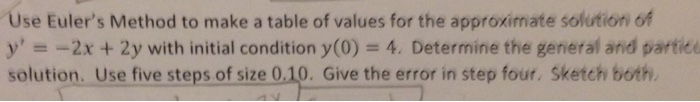Use Euler's Method to make a table of values for the approximate solution of y2x +2y with initial condition y(0) 4. Determine the general and partie solution. Use five steps of size 0.10. Give the error in step four. Sketch both. Use Euler's Method to make a table of values for the approximate solution of y2x +2y with initial condition y(0) 4. Determine the general and partie solution. Use five steps of size 0.10. Give the error in step four....

• ### Problem 1 Use Euler's method with step size h = 0.5 to approximate the solution of the IVP. 2 dy ...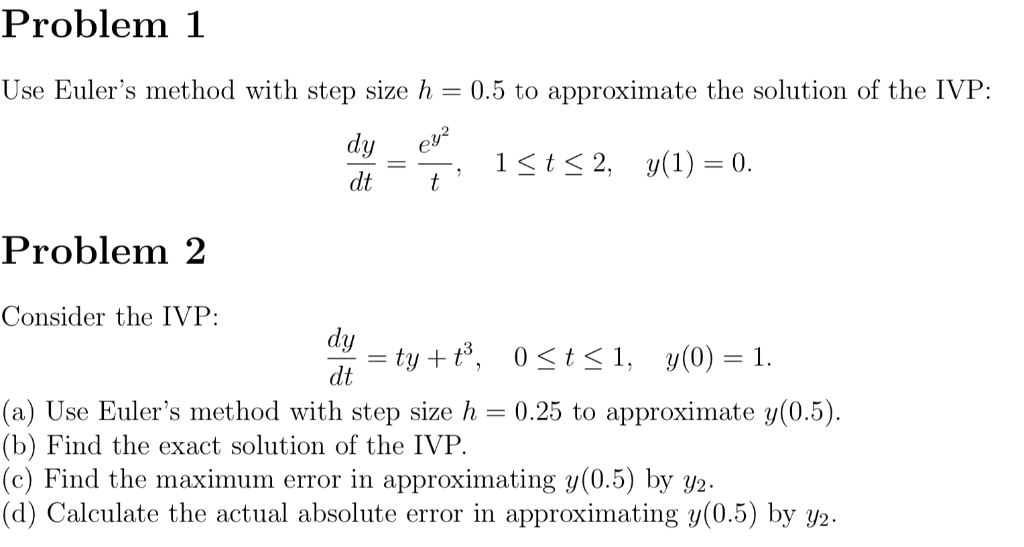Problem 1 Use Euler's method with step size h = 0.5 to approximate the solution of the IVP. 2 dy ev dt t 1-t-2, y(1) = 0. Problem 2 Consider the IVP: dy dt (a) Use Euler's method with step size h0.25 to approximate y(0.5) b) Find the exact solution of the IV P c) Find the maximum error in approximating y(0.5) by y2 (d) Calculate the actual absolute error in approximating y(0.5) by /2. Problem 1 Use Euler's method...

• ### Use Euler's method with step size h = 0.2 to approximate the solution to the initial...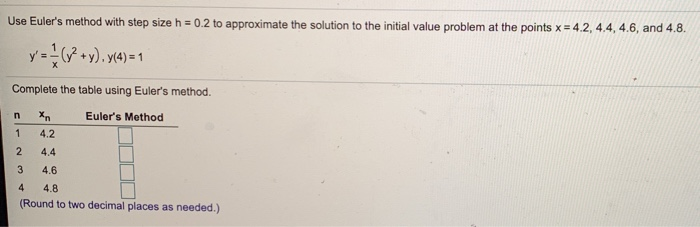Use Euler's method with step size h = 0.2 to approximate the solution to the initial value problem at the points x = 4.2, 4.4, 4.6, and 4.8. y = {(V2+y),y(4)=1 Complete the table using Euler's method. xn Euler's Method 4.2 4.4 n 1 2 2 3 4.6 4 4.8 (Round to two decimal places as needed.)

• ### Find the value of x(0.5) for the initial value problem at = thx(0)=1 using Euler's method with step size h 0.05 Find the value of x(0.4) for the coupled first order differential equations toge...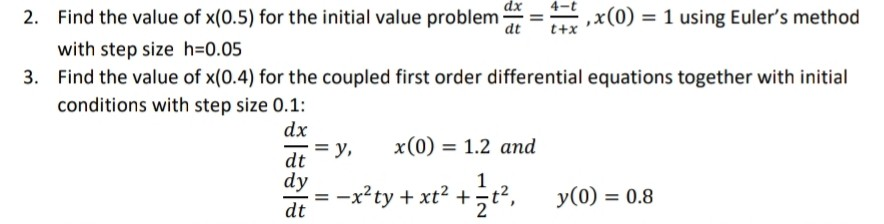Find the value of x(0.5) for the initial value problem at = thx(0)=1 using Euler's method with step size h 0.05 Find the value of x(0.4) for the coupled first order differential equations together with initial conditions with step size 0.1: 2. dt t+x 3. dx dt = y, dy dt x(0) = 1.2 and --ty +xt2 + y(o) 0.8 Find the value of x(0.5) for the initial value problem at = thx(0)=1 using Euler's method with step size h...

• ### Uestion 3. (a) 1 mark] Use Euler's method to approximate the solution of the initial-value proble...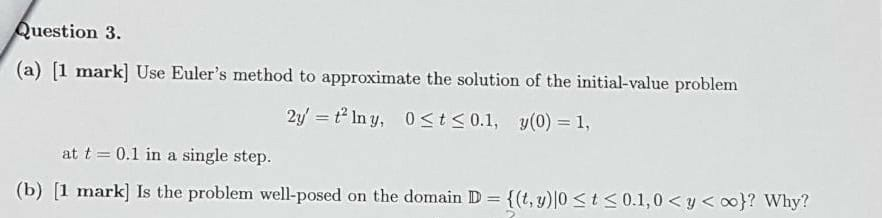uestion 3. (a) 1 mark] Use Euler's method to approximate the solution of the initial-value problem at t 0.1 in a single step. (b) [1 miark] Is the problem well-posed on the domain D {(t,y)10-K 0.1, 0 < y < ool? why? uestion 3. (a) 1 mark] Use Euler's method to approximate the solution of the initial-value problem at t 0.1 in a single step. (b) [1 miark] Is the problem well-posed on the domain D {(t,y)10-K 0.1, 0

Free Homework App